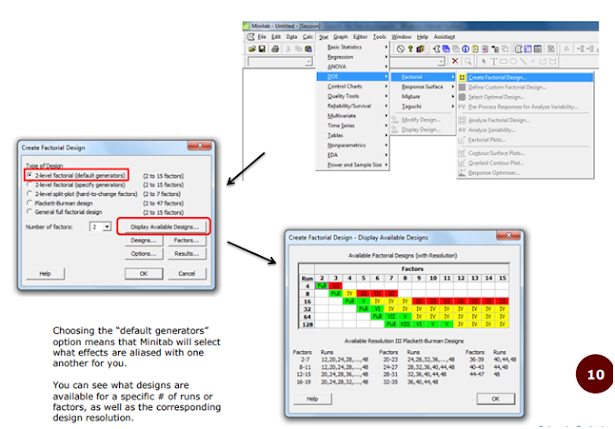# Minitab Application in Pharma | DoE | QbD

Minitab  is a software based analytical tool used in pharmaceutical industry to analyze, improve, and validate pharmaceutical processes.

#### Common Terminology Used in Minitab

Controlled Experiment: a study where treatments are imposed on experimental units, in order to observe a response

Factor: a variable that potentially affects the response

• ex. temperature, time, chemical composition, etc.

Treatment: a combination of one or more factors

Levels: the values a factor can take on

Effect: how much a main factor or interaction between factors influences the mean response

Design Space: range of values over which factors are to be varied

Design Points: the values of the factors at which the experiment is conducted

• One design point = one treatment
• Usually, points are coded to more convenient values
• ex. 1 factor with 2 levels – levels coded as (-1) for low level and (+1) for high level

Response Surface: unknown; represents the mean response at any given level of the factors in the design space.

Center Point: used to measure process stability/variability, as well as check for curvature of the response surface.

• Not necessary, but highly recommended.
• Level coded as 0

#### WHEN DO YOU USE A FACTORIAL DESIGN?

• Factorial designs are good preliminary experiments
• A type of factorial design, known as the fractional factorial design, are often used to find the “vital few” significant factors out of a large group of potential factors.
• This is also known as a screening experiment
• Also used to determine curvature of the response surface

#### Every combination of factor levels (i.e., every possible treatment) is measured.

• 2^k design = k factors, each with 2 levels, 2^k total runs
• 3^3 design = 3 factors, each with 3 levels, 3^3 = 27 total runs

Every factor effect can be estimated

Can include center points, but not necessary

2^k designs are the most popular

• High Level (+1) and Low Level (-1)

Example: 2^2 design = 4 runs

Run >  Factor A Level / Factor B Level

1 > -1 / -1

2 > -1 / +1

3 > +1 / -1

4 > +1 / +1

Full factorials can also allows factors to have different of levels
• 2^1*3^2*4^1 = 4 factors total (sum of exponents)
• One factor has 2 levels, two have 3 levels, one has 4 levels
• Total of 2*3*3*4 = 72 runs

Sometimes, there aren’t enough resources to run a Full Factorial Design. Instead, you can run a fraction of the total of treatments.

• 2^k-p design = k factors, each with 2 levels, but run only 2^k-p treatments (as opposed to 2k)
• 2^4-1 design = 4 factors, but run only 23 = 8 treatments (instead of 16)
• 8/16 = 1/2 > design known as a “½ replicate” or “half replicate”

However, not all factor effects can be estimated

• Factors are aliased with one another. In other words, factors are confounded, and you cannot estimate their effects separately.
• Ex. Suppose factors A and D are aliased. When you estimate the effect for A, you actually estimate the effect for A and D together. Only further experimentation can separate the two.
• Main effects and low order interactions are of most interest, and are usually more significant that high order interaction terms.
• So, by aliasing main effects with high order interactions, you can obtain fairly accurate estimates of the main effects.

Certain fractional factorial designs are better than others

• Determine the best ones based on the design’s Resolution
• Resolution: the ability to separate main effects and low-order interactions from one another
• The higher the Resolution, the better the design

####Note:

1. Select the factors

2. Select your design (full or fractional)

3. Select the center points (not required, but a good idea)

4. Select how many replicates for each treatment (corner points).

5. Select of blocks

Replicates
• NOT the same as repeated measurements
• Repeated measurements is when you take multiple measurements on the same unit.
• Replication is when you repeat your design a 2nd, 3rd, 4th, etc. time.
• Ex. Say you have a 2^2 design (2 factors, 4 runs) and want 3 replicates. Your experiment will have 3*2^2 = 12 runs.
• Replication will help give you more accurate effectm estimates.
• Replicates should be run at the same time as your original design (to ensure all controlled conditions are the same). If that’s not possible, consider blocking

Blocking
• Grouping together experimental units that are similar to one another – the groups are called blocks
• Blocking “reduces known, but irrelevant sources of variation between units and thus allows greater precision”

Next >